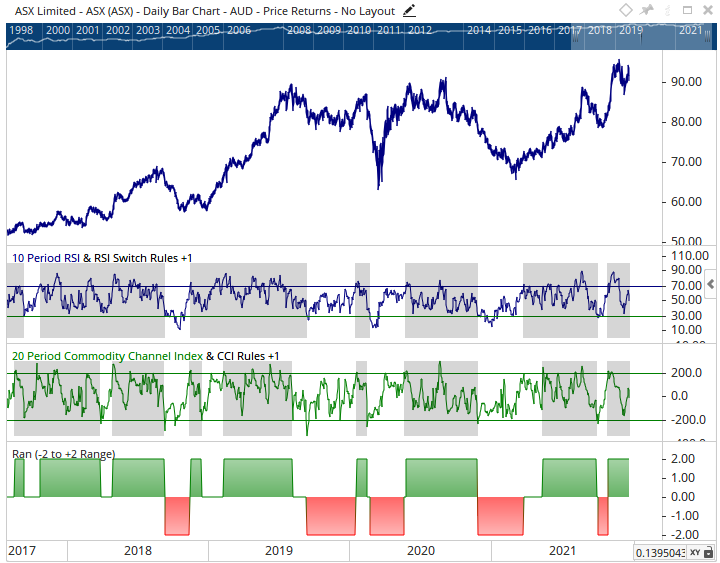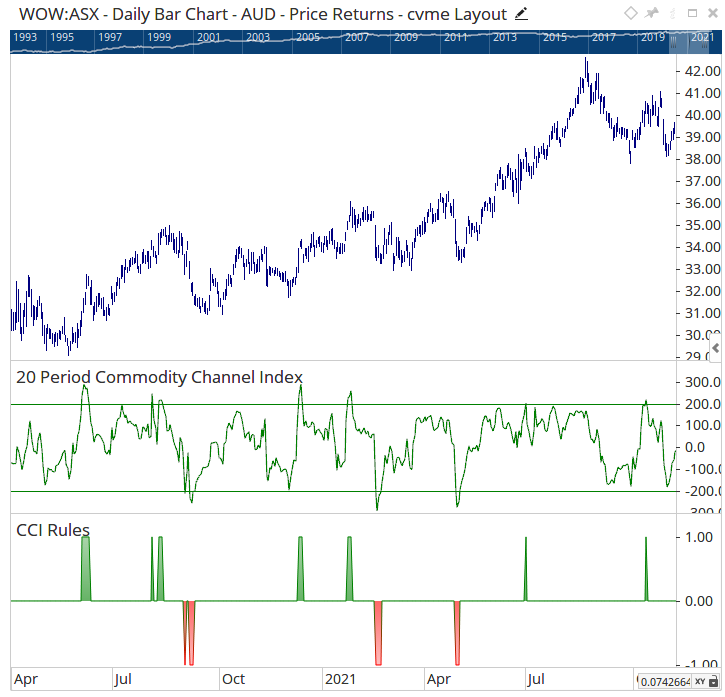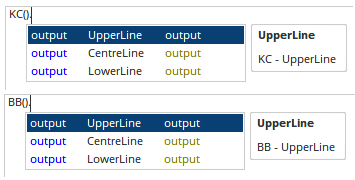Select Page

# Composite Modeling

Optuma Forums Optuma Scripting Composite Modeling

Viewing 8 posts - 1 through 8 (of 8 total)
• Author
Posts
• #66967

Hi,

I’m looking for some direction on how to script a composite model of 5 technical indicators. Each indicator would be scored +1 (pos) or -1 (neg). Examples might be:

1.      BPB (Bollinger Band) % B(20,2)

BPB(BARS=20)  (Not sure where to go after this)

2.      Normalized ROC(10)

I’m assuming this would be the proper script.

// TO CALC NORMALIZED 10 DAY ROC

//CALC IS 10 DAY ABSOLUTE PRICE CHANGE / ATR(10)

// ABS 10 DAY PRICE CHANGE

V1 = (CLOSE()-CLOSE(OFFSET=10));

// ATR(10)

V2 = ATR();

V1/V2
_____________________________

After scouring the script forum, my guess is that after all 5 indicators have been scripted the last line of the composite script would read:

V1+V2+V3+V4+V5 // To get composite score.

If someone could get me started on a proper script format, I should be able to figure out the remaining indicators (assuming that all the indicators are scripted roughly the same)

Thanks

#66975

Hi,

The first place to begin if you are new to scripting is to go over the free courses available here:

https://learn.optuma.com/optuma-courses/

These courses will give you a great foundation to start from.

Once you are familiar with scripts, you can see an example of how to build a ranking system here:

How to create a custom technical ranking system

#66979

Matthew,

I have been through all three levels of the scripting courses. I do understand the basic Boolean 1 or 0 (True or False) tests for a ranking system. However what I’m looking for is scoring system that, depending upon what the numeric value of the technical indicator is, would rank each of five technical indicators +1 or -1. The total score for the composite model would run +5 (all indicators bullish with a +1 reading) to -5 (all 5 indicators bearish with a -1).

Another example (using two of the five indicators):

RSI(10)
Rules for the indicator
If RSI(10)>= 70 then the indicator score would be +1. It would stay +1 until the indicator fell to <= 30 in which case the score would flip to -1.
If RSI (10)<= 30 then the indicator score would be -1. It would stay -1 until the indicator rose to >= 70 in which case the score would flip to +1.

CCI(20)
If CCI(20)>= 200 then the indicator score would be +1. It would stay +1 until the indicator fell to <= -200 in which case the score would flip to -1.
If CCI(20)<= -200 then the indicator score would be -1. It would stay -1 until the indicator rose to >= 200 in which case the score would flip to +1.

These are example of the basic parameters of each indicator. It seems to me that such a script would contain elements of an IF Statement and or perhaps a Switch(). I’m just unsure of how to construct the script for each indicator and sum them up. Any clues would be appreciated.

P.S. I know this model can be created. What I’m looking to build is a variation on a model that Arthur Hill had created using Optuma. Tip of the hat to Arthur.

#66981

You would need to use both the Switch() and IF() functions.

First setting up the two trigger rules as booleans (RSI Crosses above 70 and RSI Crosses below 30)…

Then wrapping those in a Switch() function…

Then using the IF() to set the values of +1 and -1 …

These steps would repeated for all the tool rules you needed to build.  CCI complete example:

The final line of the script would then add all the “RESx” variables together…

The final result would look like this (bottom view tool)…###### 1 user thanked author for this post.Andrew
#67019

Hi,

After further research on this model. It appears that in addition to the scoring parameters of:
1.            If indicator value crosses above +200 = +1

2             If indicator value crosses below -200 = -1

There is a third parameter where:
3.            If indicator value is < +200 and > -200 then = 0

Here is the script from just one indicator to use as an example. This code works fine for defining parameters 1 &2.

The trouble comes when I try to introduce parameter #3. I’ve tried a nested if statement that looks like this:

No matter how I write the Nested if statement where IF(Test Condition,Then, Else) I receive an invalid Script statement from the Script manager. Is the Switch Function necessary? Any clues would be appreciated.

TIA

#67032

Hi,

In the original post you stated:

If RSI(10)>= 70 then the indicator score would be +1. It would stay +1 until the indicator fell to <= 30 in which case the score would flip to -1.

This rule is why the Switch() function is needed, there were only 2 outcomes and one always needed to be true.

What you are describing now is a different set of rules with 3 outcomes.  For the CCI that looks to be:

• CCI > 200
• CCI < -200
• CCI >-200 and < 200

Nested IFs would be the way to do this, SWITCH() is no longer required.

See article here: https://help.optuma.com/kb/faq.php?id=1085

CCI Example:

Chart Example…###### 1 user thanked author for this post.Andrew
#67053

One more thing:

Hi,

Working on a different model that includes Keltner channels and Bollinger Bands. I need to set bull +1 and bear -1 conditions on crosses above and below the upper and lower band and channel lines. Here’s what I have now (I pretty sure it incorrect as the compiler won’t accept it).

My question is, is there dot notation that can be used to delineate the upper and lower channels and bands or is there another way?

#67062

Hi,

Yes, both the Keltner Channel and Bollinger Band script functions include dot notations to select the value you wish to reference (Upper, Central or Lower lines)…###### 1 user thanked author for this post.Andrew
Viewing 8 posts - 1 through 8 (of 8 total)
• You must be logged in to reply to this topic.